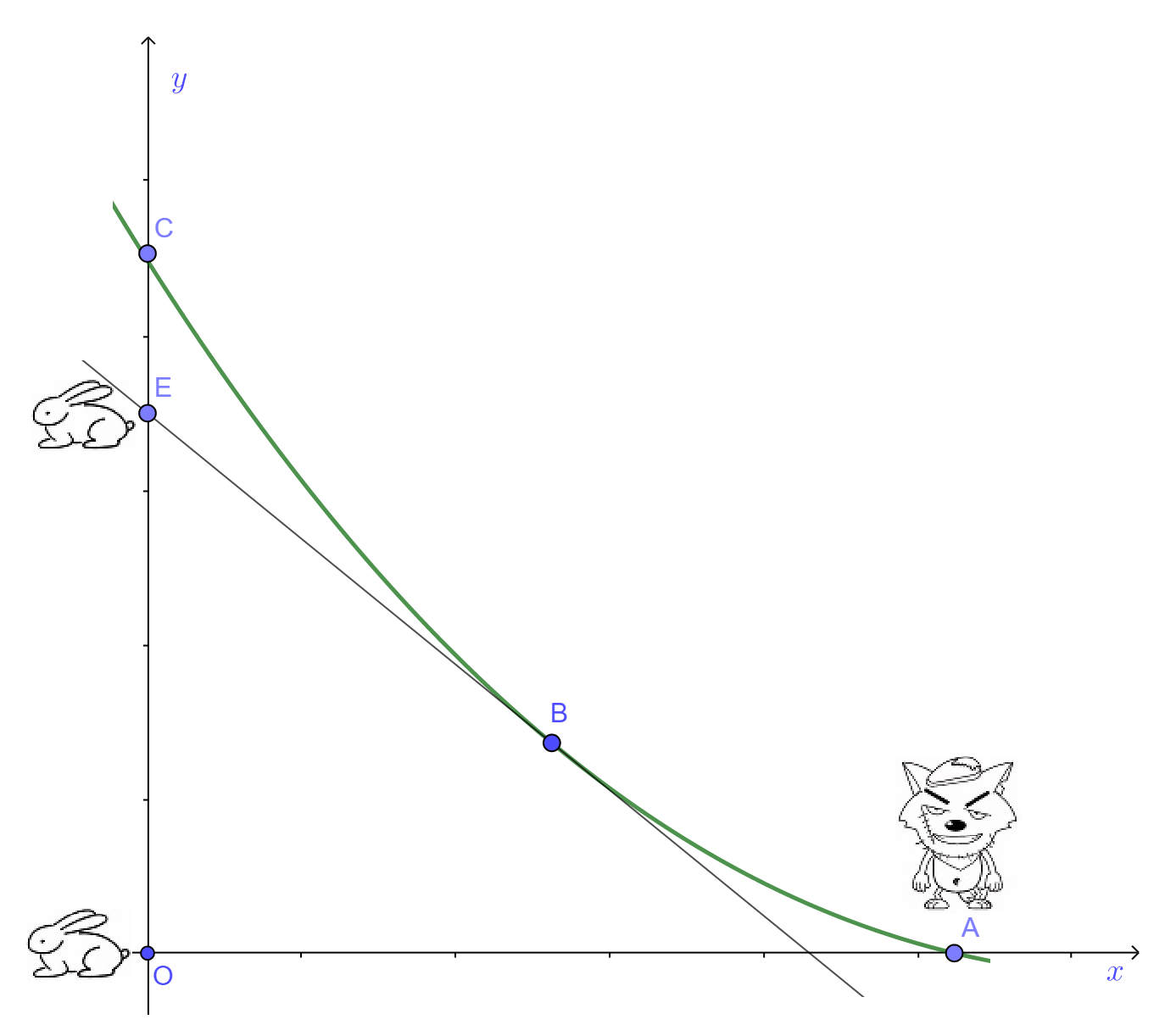安卓手机扫描二维码安装App﻿$y=\dfrac{x^{1+c}}{2{a^c}(1+c)}-\dfrac{{a^c}{x^{1-c}}}{2(1-c)}+\dfrac{ac}{1-c^2}$﻿

﻿$x=0$﻿

﻿$a=100$﻿

﻿$c=\dfrac{1}{2}$﻿

﻿$x$﻿﻿$a$﻿﻿$c$﻿ 代入方程，解得的﻿$y$﻿ 即是狼追上兔子时兔子所跑的距离OC，所以兔子洞距离兔子的起点O的距离必须小于OC才不致于被捕到。请代入﻿$x$﻿﻿$a$﻿﻿$c$﻿，用计算器计算幂。 计算出OC的距离。苹果手机扫描二维码安装App